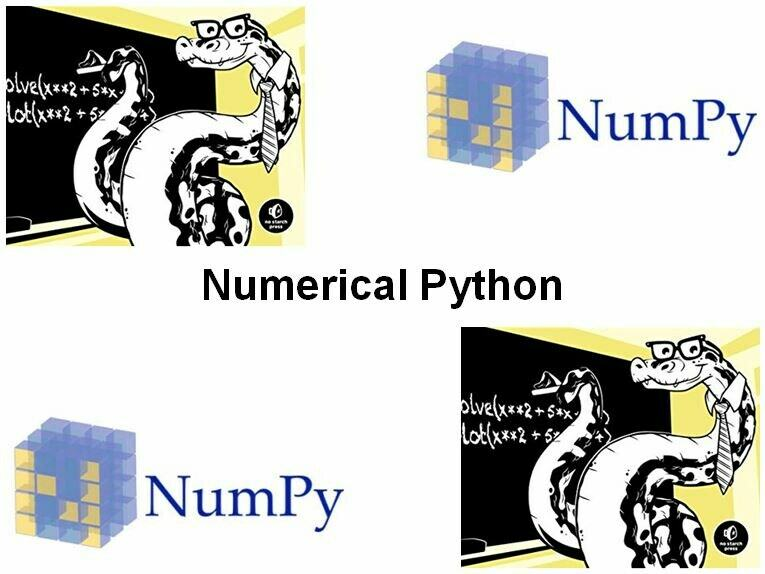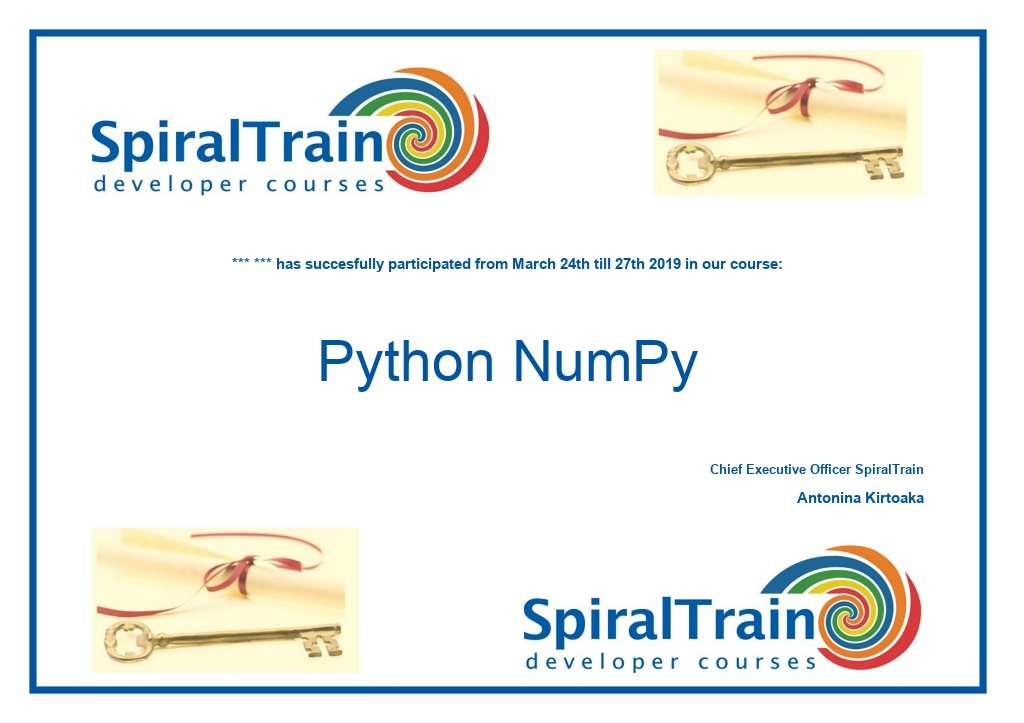# Course Python NumPy

In the course Python NumPy the Python packages NumPy en MatplotLib are discussed. These Python add-on libraries are very useful for the creation of data analysis and data processing applications.

Region:
1 day
€ 699
• Content
• Training
• Modules
• General
General
• Reviews
• Certificate
• ## Course Python NumPy : Content

### Overview NumPy and SciPy

The course starts with an overview of NumPy and its sister library SciPy and how we can install these libraries.

### NumPy ndarray

Next the NumPy's ndarray object and its methods are discussed. Attention is paid to many different array manipulation techniques. These methods process large data sets very efficiently.

### Matrix Handling

Next matrix handling with Numpy is treated and attention is paid to special routines for ordening, searching and comparing data in matrices.

### MatPlotLib

Finally the MatplotLib library is discussed. This library is closely integrated with NumPy and SciPy and this makes it a very powerful tool to create and plot complex figures. The course uses real world examples to visualize of one- and two dimensional data.

• ## Course Python NumPy : Training

### Audience Python NumPy Course

The course Python NumPy is intended for scientists and Big Data analysts who want to use Python with NumPy and MatPlotLib for data analysis and data processing.

### Prerequisites Course Python NumPy

To participate in this course prior knowledge of Python programming is necessary. Knowledge of numerical methods is beneficial for the understanding.

### Realization Training Python NumPy

The theory is dealt with on the basis of presentation slides. The concepts are illustrated with demos. The theory is interspersed with exercises. The course times are from 9.30 to 16.30.

### Certification Python NumPy

The participants receive an official certificate Numerical Python after succesful completion of the course.• ## Course Python NumPy : Modules

### Module 3 : Matrices

What is NumPy?
What is SciPy?
Installing NumPy
NumPy array object
Selecting elements
NumPy numerical types
Data type objects
dtype constructors
dtype attributes
Onedimensional slicing and indexing
Multidimensional slicing and indexing
Array comparisons
any(),all(), slicing, reshape()
Manipulating array shapes
Stacking and Splitting arrays
Converting arrays
Methods of ndarray
Clipping arrays
Compressing arrays
Views versus copies
ravel(),flatten(),transpose()
Missing values
Handling NaNs
nanmean(), nanvar() and nanstd()
File I/O
mean() function
Value range
Dates
Correlation
Smoothing
full() and full_like() functions
Working with Matrices
ufuncs
Creating matrices
Universal functions
Arithmetic functions
Modulo operation
Fibonacci numbers
Bitwise functions
Comparison functions
Fancy indexing
at() method
Inverting matrices
Finding eigenvalues
Singular value decomposition
Pseudo inverse
Determinants

### Module 5 : Plotting with MathplotLib

Sorting
partition() function
Complex numbers
Searching
Array elements extraction
Assert functions
Almost equal arrays
Equal arrays
Ordering arrays
Object comparison
String comparison
Floating point comparisons
Unit tests
Simple plots
Plot format string
Subplots
Histograms
Logarithmic plots
Scatter plots
Fill between
Legend and annotations
Threedimensional plots
Contour Plots
Transformations
Animation
Projections
• ## Course Python NumPy : General